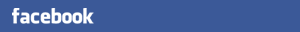# 年輕用語知多少？　知道TPL是什麼意思嗎？不知道就落伍了！

• A
• A+
• A++
2016-10-24(圖/取自網路)(圖/取自網路)

56 = 無聊　043 = 你是誰　184 = 一輩子　469 = 死老猴　530 = 我想你　584 = 我發誓　748 = 去死吧　770 = 親親你

837 = 別生氣　865 = 別惹我　880 = 抱抱你　04551 = 你是我唯一　04592 = 你是我最愛　0451625 = 你是否依然愛我

0564335 = 你無聊時想想我　2U = To You　4U = For You　AV8D = Everybody　SPK2U = Speak to you　AKS =會氣死

ATOS =會吐死　BMW =大嘴巴(Big Mouth Wowan)　BPP = 白泡泡(台語：皮膚很白)　CD = 死豬　CBA = 酷斃啦　CKK = 死蹺翹

FDD = 肥嘟嘟　GND = 很英俊 (台語）　LOA = 老芋仔　MGG = 醜斃了(台語：醜吱吱)　OBS = 歐巴桑　OGS = 歐吉桑

OKE = 我可以　OIC = 喔，了解 (Oh, I see)　PDG = 皮在癢（台語）　PMP = 拍馬屁　RUOK = Are You OK？　SBB = 三八八

SDD = 水噹噹　SPP = 聳斃了　SYY = 爽歪歪　TMD = 他媽的　TPL = 土芭樂　UKLM = 幼齒辣妹　醬 = 這樣　釀 = 那樣

【101傳媒／整理報導】

-------------------------------------------------------

• 本文評論：